Create a new printable
Answer key also includes questions
Answer key only gives the answers
No answer keyGeometry
Math Worksheets

Sample - Click above to make a new math worksheet (PDF).
 Name _____________________________Date ___________________
Circumference
To the nearest tenth, find the circumference. Use 3.14 for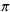.

1.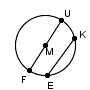FM
=  31.9 in
2.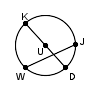DK
=  12.17 cm
3.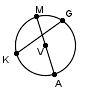AM
=  86.348 ft
4.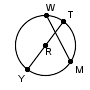TR
=  15.29 mm
5.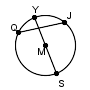SY
=  29.155 in
6.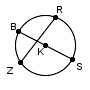KB
=  10.6 ft
7.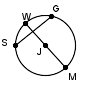JM
=  38.4 cm
8.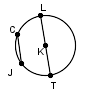TL
=  9.53 mm
9.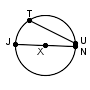NJ
=  48.28 cm
 10 * This is a pre-made sheet.Use the link at the top of the page for a printable page.
11.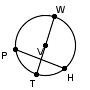TW
=  21.669 mm
12.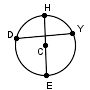EC
=  14.78 ft
13.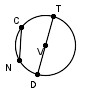DT
=  43.61 in
14.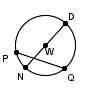DN
=  40.6 ft

 Answer Key

Sample
This is only a sample worksheet.

Create a new printable
Answer key also includes questions
Answer key only gives the answers
No answer keyGeometry
Math Worksheets